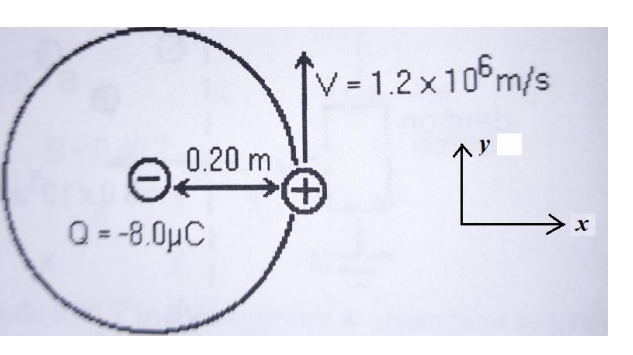# Question An ion of mass m and charge + e is in circular motion around a point charge Q with –8.0 microCpulomb charge. The radius of the orbit is 0.20 m and the speed of the orbiting ion is 1.2x106 m / s. A Uniform magnetic field is present and is perpendicular to the plane of the orbit. Magnetic force on the ion it is equal to the electric force in magnitude and direction at all points in the orbit. With This information will determine: 5. The magnetic field vector. 6. The mass of the ion is: 7. The radial acceleration is: V = 1.2 x 106m/s AY 0.20 m © # O - -8.0μο х```An ion of mass m and charge + e is in circular motion around a point charge Q with
–8.0 microCpulomb charge. The radius of the orbit is 0.20 m and the speed of the orbiting ion is 1.2x106 m / s. A
Uniform magnetic field is present and is perpendicular to the plane of the orbit. Magnetic force
on the ion it is equal to the electric force in magnitude and direction at all points in the orbit. With
This information will determine:
5. The magnetic field vector.
6. The mass of the ion is: